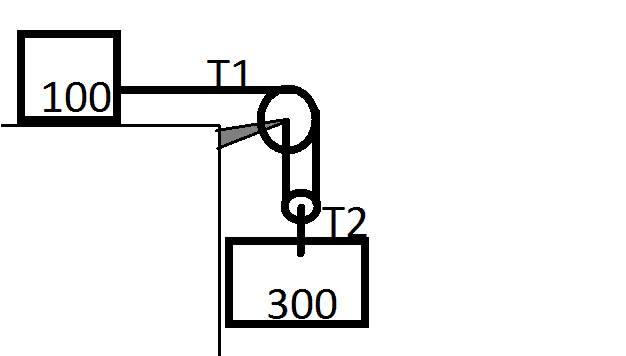# Dynamics: 2 block problem

## Homework Statement

Assuming no friction is on the plane or the pulleys and the pulley and rope have negligible mass... Determine the acceleration of each block and the tension in each cord. Block A weighs 100kg, Block B weighs 300kg.ΣF = ma
ma = 100kg
mb = 300kg

## The Attempt at a Solution

ΣFx = max = T1 = 100ax
ΣFy = may = W - T2 = 2943kg - T2 = 300ay
T1=2T2

I know that I have the relationships right, but I don't know exactly how to extract them...

What is the relationship between ax and ay? It is constrained motion, so you relate the two accelerations.

I think I solved it this way based off of what my professor drew on the board but I need some clarity because I'm not quite sure how this can be...
if we assume:

ax = 2ay
^My professor's hint

then we have 4 relations and 4 unknowns. So:

T1 = 2T2
100ax = 2[2943 - 300ay]
50ax = 2943 - 300ay
ax = 2ay
50*2ay = 2943 - 300ay
400ay = 2943

ay = 7.3m/s^2
ax = 14.8 m/s^2

**Edit**
This is an incorrect answer. The correct answer is 8.4m/s^2 for ax which means somewhere my answer is almost 2 times larger.

Last edited: